# The Cantellated 120-cell

The cantellated 120-cell is a uniform polychoron of the 120-cell/600-cell family. Its surface consists of 1920 cells (120 rhombicosidodecahedra, 600 octahedra, 1200 triangular prisms), 9120 polygons (4800 triangles, 3600 squares, 720 pentagons), 10800 edges, and 3600 vertices.

It may be constructed by radially expanding the octahedral cells of the rectified 600-cell outwards, which causes the icosahedral cells to become rhombicosidodecahedra, and filling the gaps with triangular prisms.

## Structure

We shall explore the structure of the cantellated 120-cell by using its parallel projection into 3D, centered on one of the 120 rhombicosidodecahedral cells.

### First Layer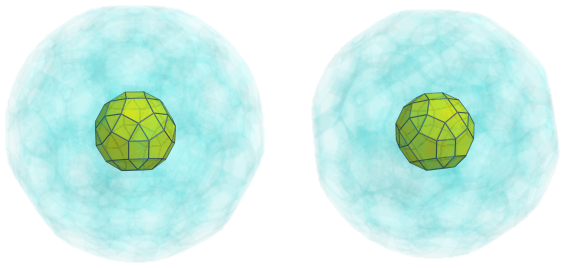The above image shows the closest rhombicosidodecahedral cell to the 4D viewpoint. For clarity, we have omitted edges and vertices that do not lie on this cell, and have rendered all other cells with a light transparent color.

The triangular faces of the nearest rhombicosidodecahedron are joined to 20 octahedra, as shown next: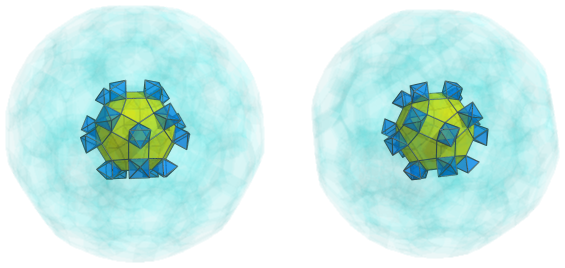These octahedral cells are connected to each other by 30 triangular prisms, which touch the central rhombicosidodecahedron's square faces: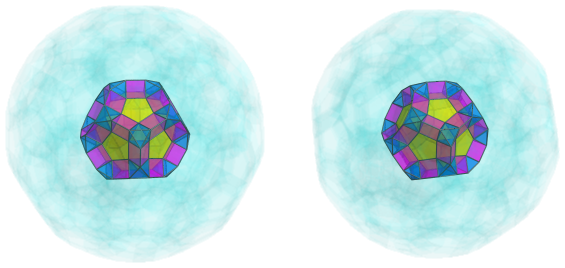### Second Layer

Another 20 triangular prisms are connected to the octahedra, the beginnings of a network of triangular prisms and octahedra that permeates the surface of the cantellated 120-cell: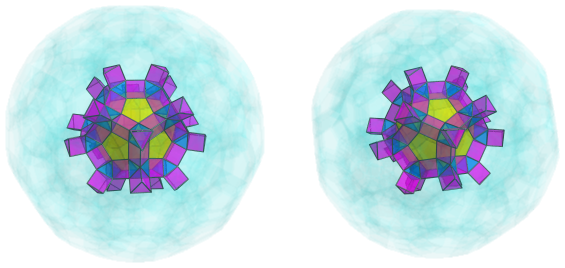These triangular prisms are capped by 20 more octahedra: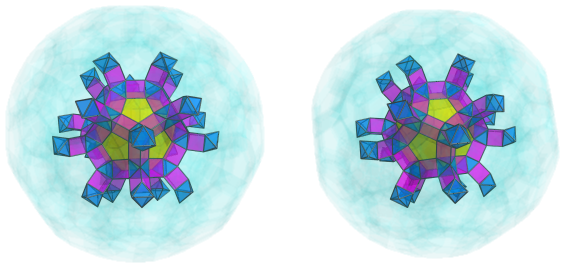Touching the pentagonal faces of the central cell are 12 other rhombicosidodecahedral cells that fit into the framework of triangular prisms and octahedra: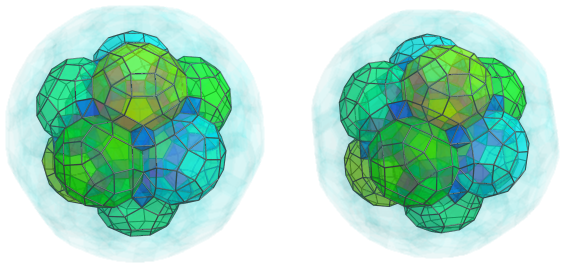These cells are girded by more triangular prisms and octahedra in the framework: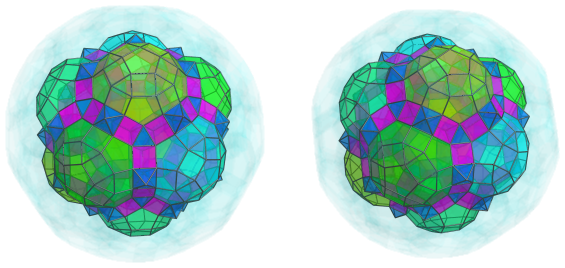There are 60 more triangular prisms and 30 more octahedra here.

### Third Layer

The framework of triangular prisms and octahedra continues over the square and triangular faces of the 12 rhombicosidodecahedra, as shown next: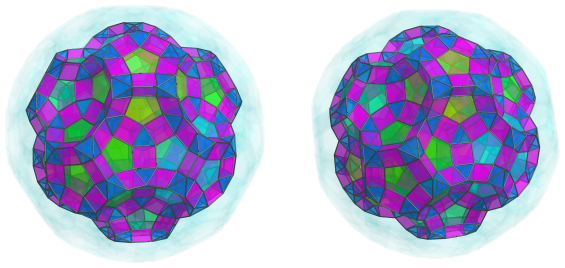There are 60+60=120 more triangular prisms here, as well as 60 more octahedra. The 60 octahedra, of course, have another 60 triangular prisms attached to them: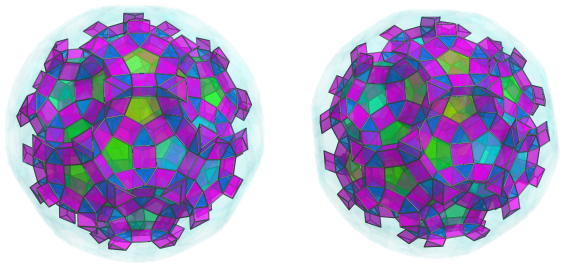As is clearly seen, these triangular prisms and octahedra trace out the outlines of more rhombicosidodecahedral cells. We show another 20 of the latter below: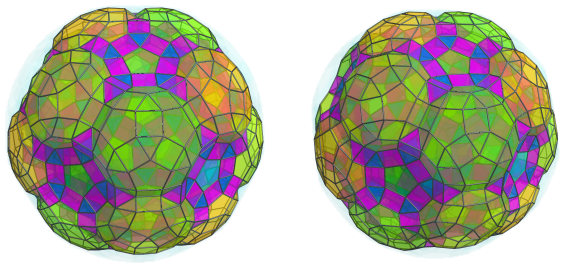The remaining gaps are filled in by another 12 rhombicosidodecahedra: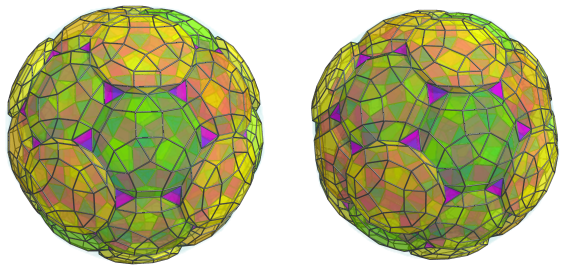### Fourth Layer

The exposed triangular faces of the triangular prisms from the previous layer are capped by 60 more octahedra: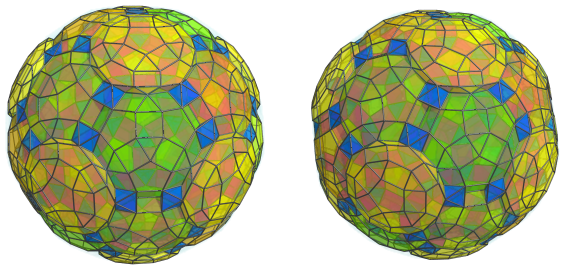These octahedra are bridged to each other by more triangular prisms and octahedra which gird the rhombicosidodecahedral cells: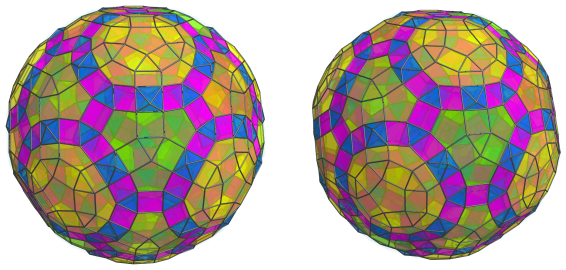There are a total of 60+60+30=150 more triangular prisms and 60 more octahedra here. These 60 octahedra have another 120 triangular prisms connected to them: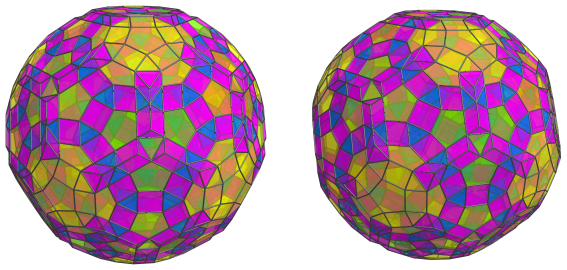Finally, 60 of these 120 triangular prisms are capped by another 20 octahedra: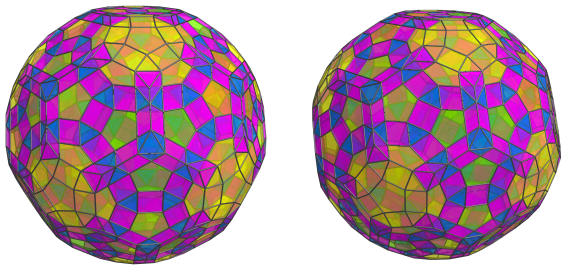These are all the cells on the near side of the cantellated 120-cell. Past this point, we reach the cells on the limb, or “equator”, of the polytope.

### The Equator

There are 30 rhombicosidodecahedral cells that lie on the equator of the cantellated 120-cell, as shown below: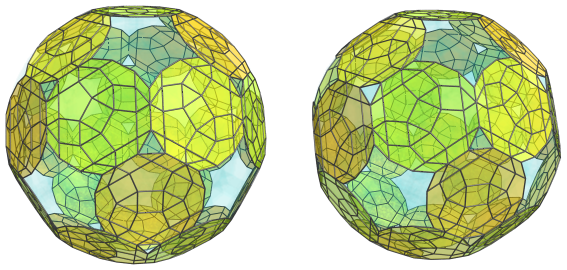For clarity, we have omitted the other cells that we have seen so far.

These rhombicosidodecahedra appear to be flat, because they are being seen from a 90° angle. In 4D, they are perfectly uniform.

There are also 60 octahedral cells on the equator: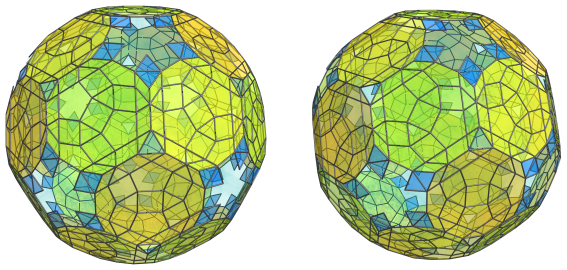Since these octahedra are being seen from a 90° angle, they appear flattened into rhombuses. Between them are 60 triangular prisms: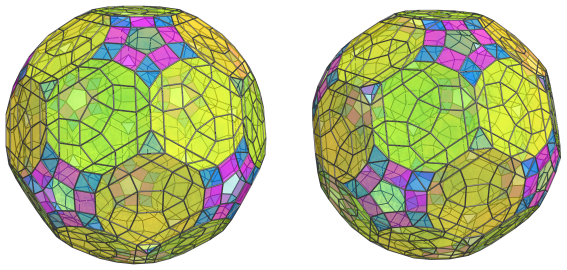These triangular prisms, being seen from a 90° angle, have been foreshortened into rectangles. The edges of the rectangles that touch the rhombicosidodecahedra are the images of one of their square faces.

There are another 20 triangular prisms that sit between each triplet of rhombicosidodecahedra: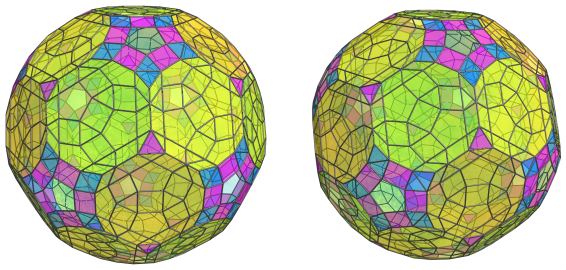These triangular prisms connect the last 20 octahedra on the near side of the cantellated 120-cell with their counterparts on the far side. Due to their different orientation with respect to the previous triangular prisms, they have been foreshortened into triangles instead.

These are all the cells that lie on the equator of the cantellated 120-cell. The remaining pentagonal gaps are not the images of any cells; they are where 12 of the rhombicosidodecahedral cells on the near side touch their counterparts on the far side.

Past this point, we reach the far side of the polychoron, where the arrangement of cells mirrors the arrangement we've seen on the near side.

### Summary

The following table summarizes the cell counts for the cantellated 120-cell:

Region Layer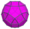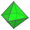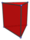Near side 1 1 20 30
2 12 20 + 30 = 50 20 + 60 = 80
3 20 + 12 = 32 60 120 + 60 = 180
4 0 60 + 60 + 20 = 140 150 + 120 = 270
Subtotal 45 270 560
Equator 30 60 60 + 20 = 80
Far side 4 0 140 270
3 32 60 180
2 12 50 80
1 1 20 30
Subtotal 45 270 560
Grand total 120 600 1200

## Coordinates

The coordinates of the cantellated 120-cell, centered on the origin and having edge length 2, are all permutations of coordinates and changes of sign of:

• (0, 0, 2φ3, 4φ2)
• (1, 1, φ3, 3φ3)
• (1, 1, 3+4φ, 3+4φ)
• (2φ, 2φ2, 2φ3, 2φ3)
• 3, φ3, 1+4φ, 3+4φ)

along with all even permutations of coordinates and all changes of sign of:

 (0, 1, 4+5φ, 1+3φ) (0, φ, φ5, 1+4φ) (0, φ2, 3φ3, 2+φ) (0, φ3, 2+5φ, 3φ2) (1, φ2, 2+5φ, 2φ3) (1, φ2, 4+5φ, 2φ2) (1, 2φ, φ5, φ4) (1, φ4, 2φ3, 3φ2) (φ, φ2, 2φ, 3φ3) (φ, 2φ2, 3+4φ, 3φ2) (φ2, 2φ, 4+5φ, φ3) (φ2, 2+φ, 3+4φ, 2φ3) (φ2, φ3, 4φ2, φ4) (φ2, φ4, 1+4φ, 2φ3) (2φ, 1+3φ, 3+4φ, φ4) (2+φ, φ3, φ5, 2φ2) (φ3, 2φ2, 1+3φ, 2+5φ)

where φ=(1+√5)/2 is the Golden Ratio.

Last updated 02 Feb 2023.